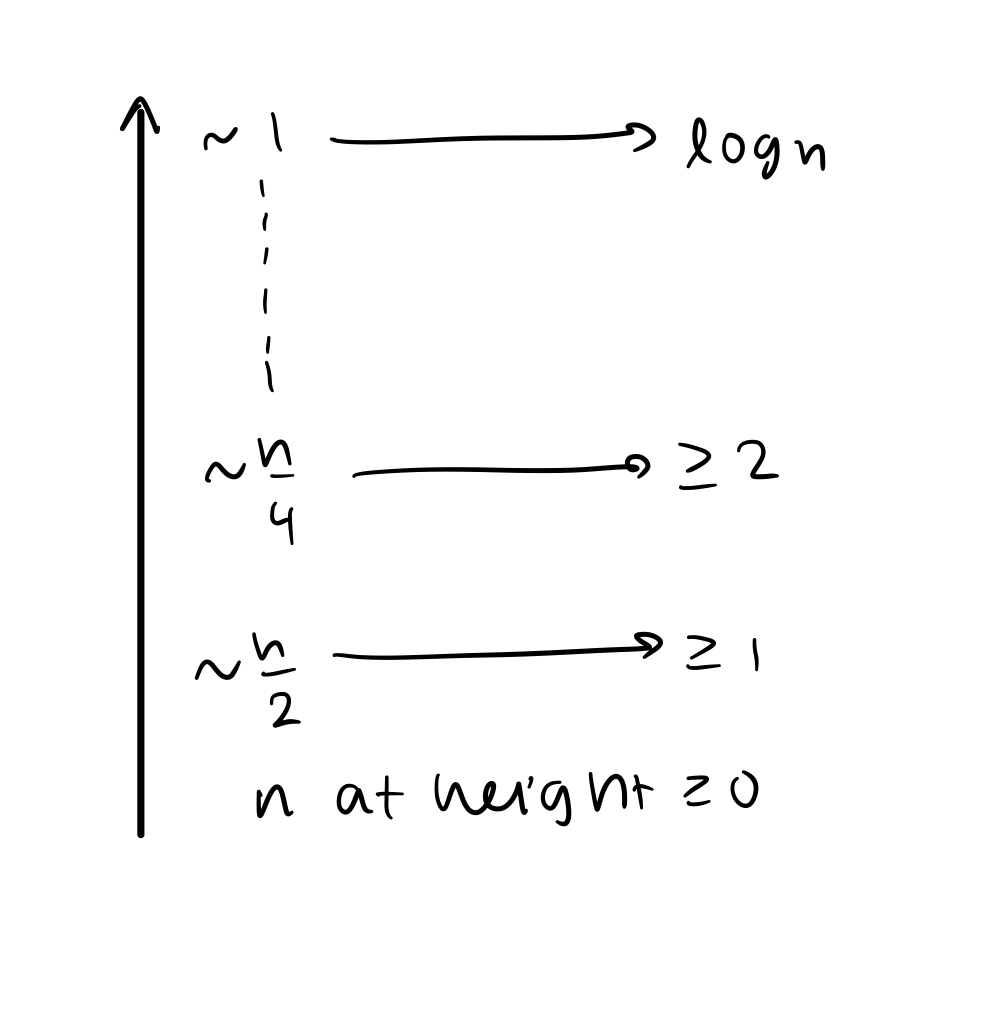Scribes:

• Tanmai Pathak

1. Skiplists

Announcements

• Quiz 03 (due Tuesday)
• Assignment 5 posted tonight (no data structures!)

Last Time

Sorted Sets: S = {x_0, x_1, … , x_n} where x_0 < x_1 < … < x_n

1. Linked list of nodes: all operations are O(n)
2. Sorted array: add/remove are O(n), find is O(logn) because of binary search
3. Unbalanced BST: all operations O(h)
• h = height of tree
• depends on order of operations
• h <= n-1 (always)
• random adds h is typically O(logn)
4. Balanced BST (AVL tree): operations are still O(h) but maintain h = O(logn) which is always true

Going Forward

Randomized data structures

• Introduce randomness
• Probabilistic guarantees
• good behavior, most of the time independent of what user is doing
• Often: simpler solutions than deterministic

Skiplists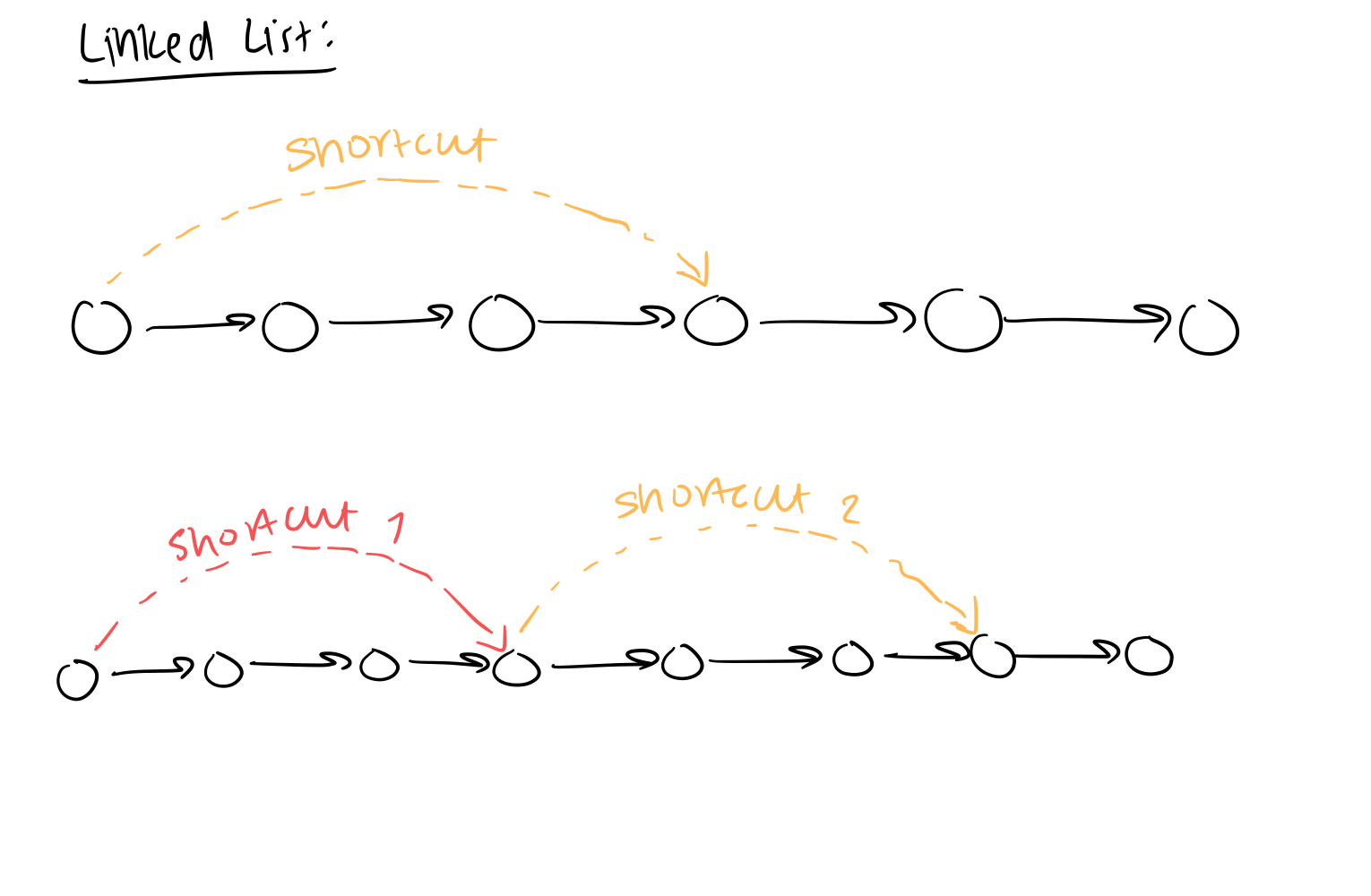• Adding shortcuts might improve search time
• When adding and removing, the shortcuts have to be changed
• might be really expensive to maintain the shortcuts

Idea:

• Associate height to each node
• if node v has a height h, store a shortcut to next nodes of height at least h, h-1, …, 0
• Sentinel node
• first
• doesn’t store value
• h = max heigh of all nodes
• More formally: each node v stores an array of h(v) “next” nodes next[i] = shortcut at height i
• only applies to nodes that also have at least the same height as the nodes being pointed to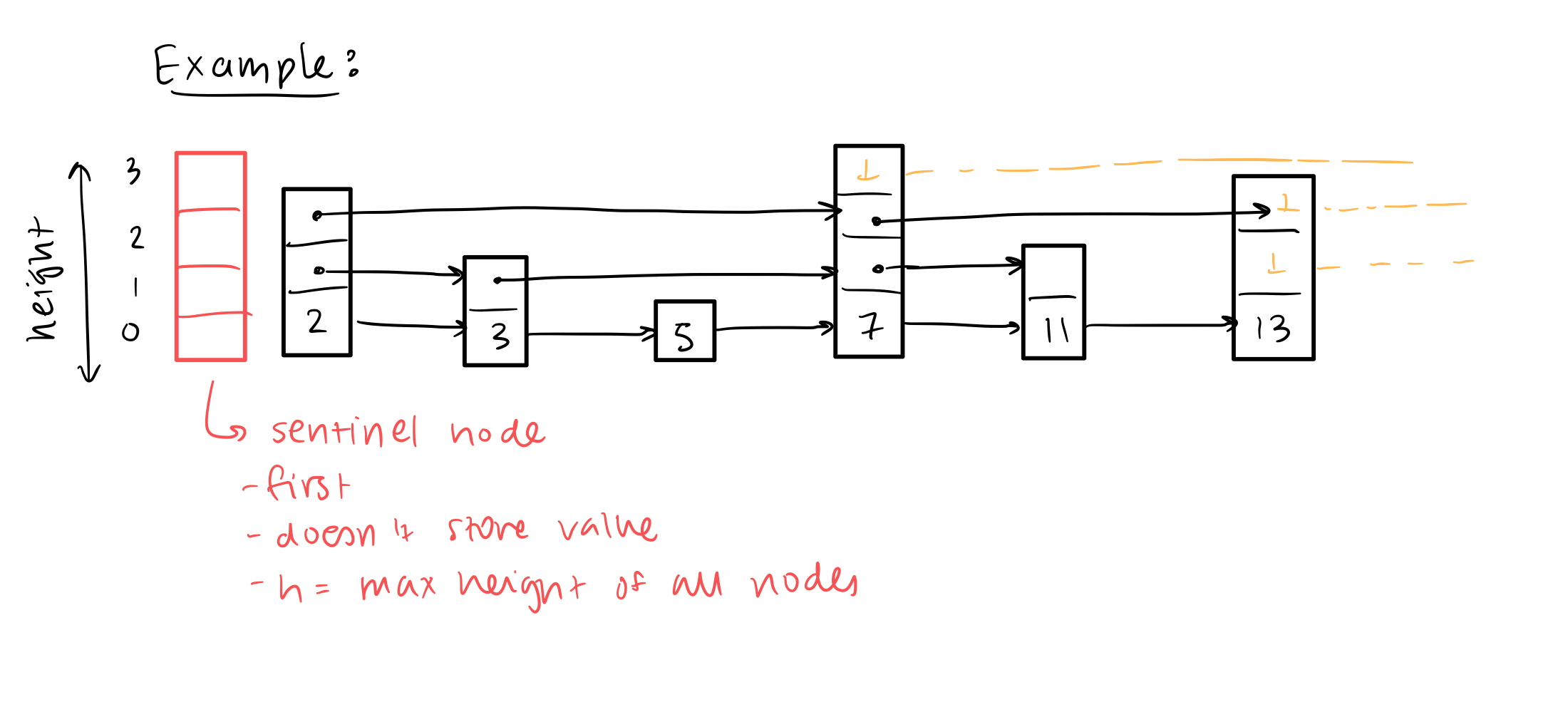How to find(x)?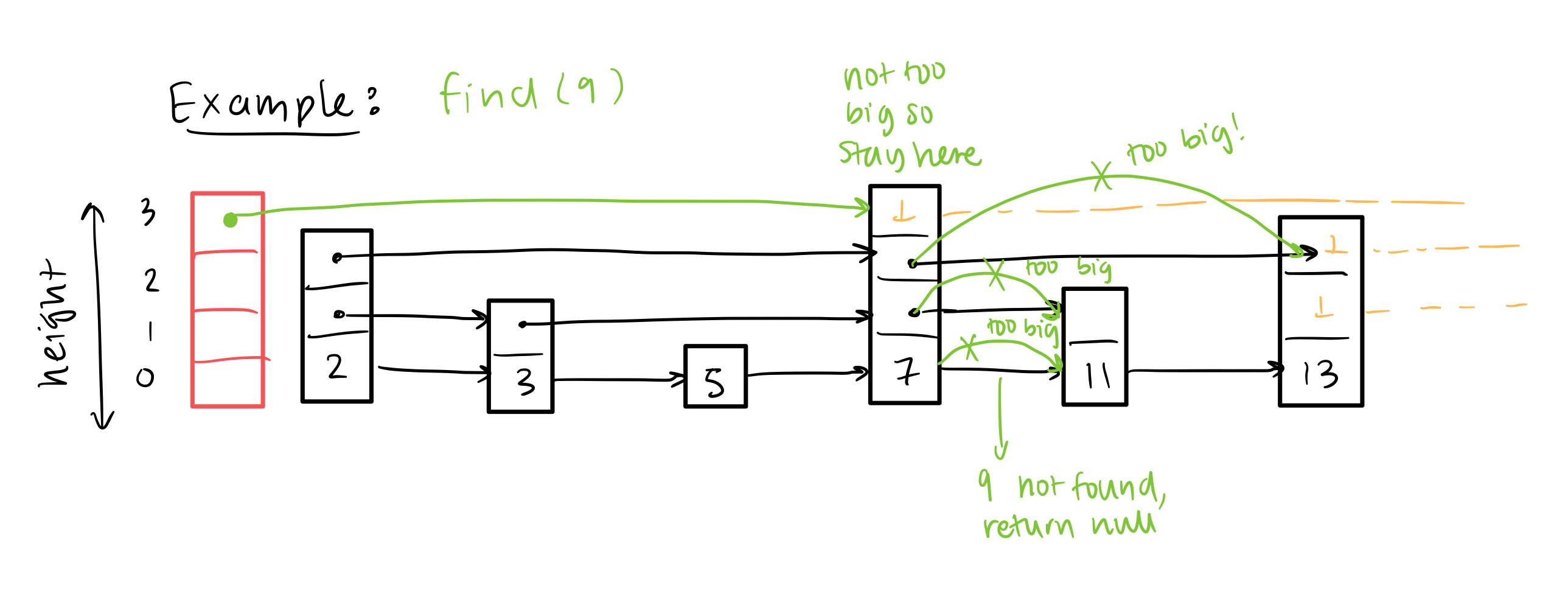Generally:

1. Start at v = setinel node
2. h = max height
3. Find next node (w) at height >= h (next[h])
• if value of w = x, return value of w
• if value of w < value of x, update v = w
• if value of w > value of x, decrease height and repeat step 3
• if h < 0, return null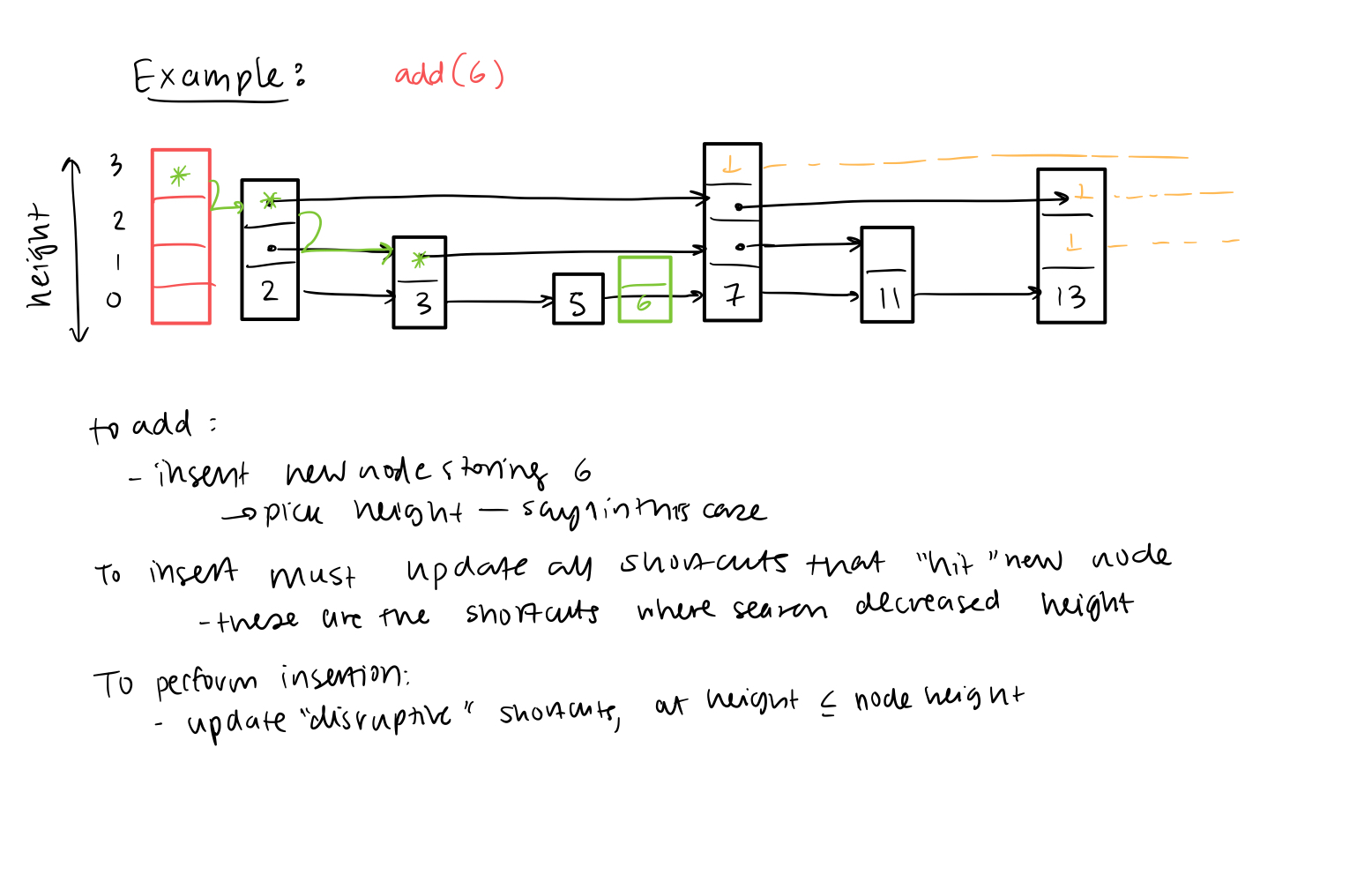How to remove(x)?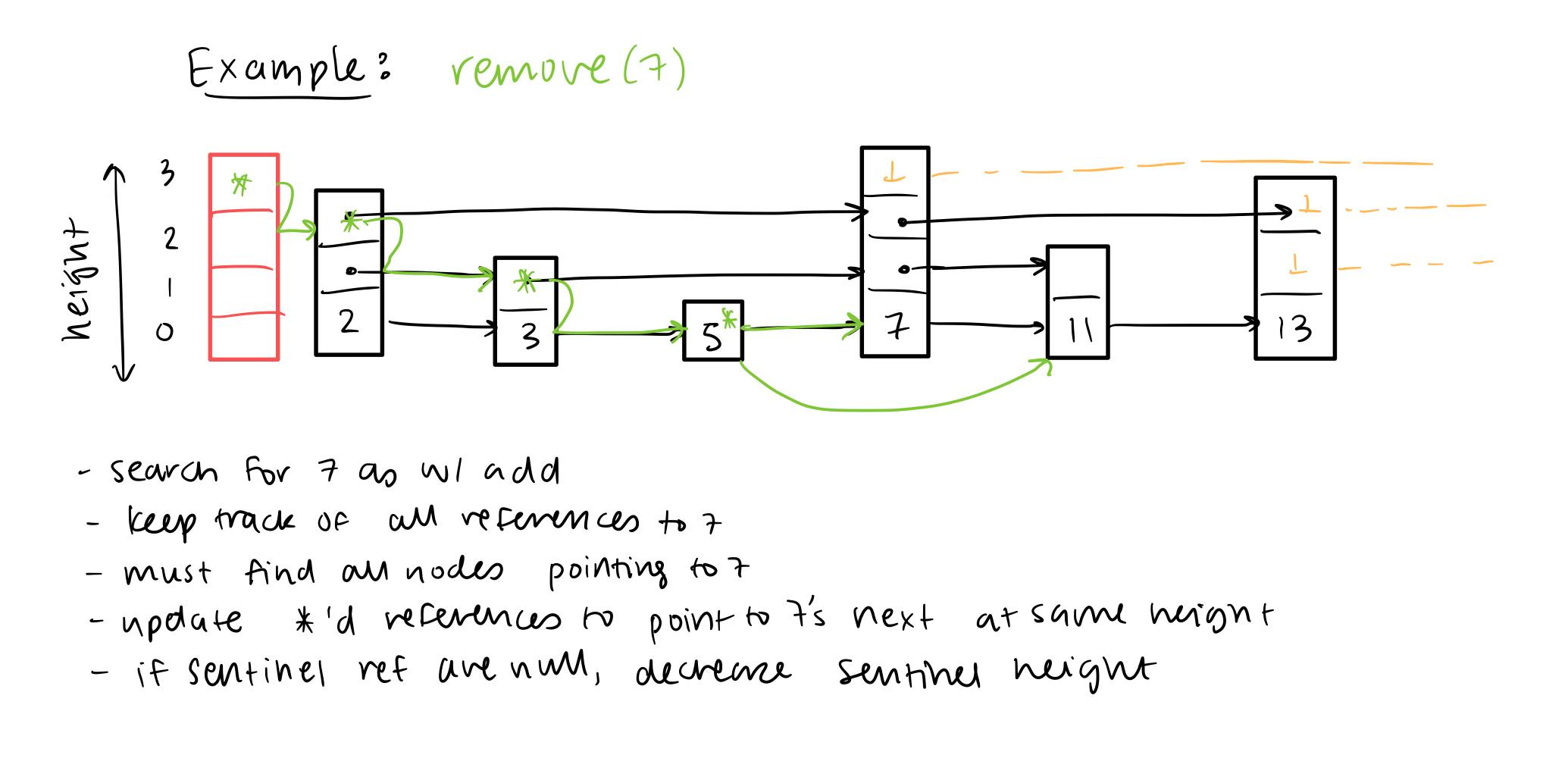Lingering Question

How to choose height of new nodes?

• What do we want?
• hierarchical structure:
• if “balanced BST - like”
• one tallest node in the middle - height = h
• two (or few) nodes at height h - 1
• fourish at height h - 2
• n at heigth >= 0
• Random process to generate height:
• flip coins: each flip is H/T (equally likely)
• h = # of T before first H
• Then:
• about n have height 0
• about 1/2n have height >= 1
• about 1/4n have height >= 2
• about 1/8n have height >= 3